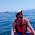Support us .Net Basics C# SQL ASP.NET ADO.NET MVC Slides C# Programs Subscribe Buy DVD

### Web API attribute routing constraints

Suggested Videos
Part 29 - ASP.NET Web API facebook authentication
Part 30 - Attribute routing in ASP.NET Web API 2
Part 31 - RoutePrefix attribute in Web API

In this video we will discuss Attribute Routing Constraints in ASP.NET Web API. Let's understand these constraints with an example.

Consider the following StudentsController.

[RoutePrefix("api/students")]
public class StudentsController : ApiController
{
static List<Student> students = new List<Student>()
{
new Student() { Id = 1, Name = "Tom" },
new Student() { Id = 2, Name = "Sam" },
new Student() { Id = 3, Name = "John" }
};

[Route("{id}")]
public Student Get(int id)
{
return students.FirstOrDefault(s => s.Id == id);
}
}

If we navigate to /api/students/1, Get(int id) action method is mapped to the URI and we get the details of the student whose id is 1 as expected.

In addition to retrieving student by Id, we also want to retrieve student by "name". So let's add another Get() method as shown below. Notice the name of the parameter is name and it's type is string.

[RoutePrefix("api/students")]
public class StudentsController : ApiController
{
static List<Student> students = new List<Student>()
{
new Student() { Id = 1, Name = "Tom" },
new Student() { Id = 2, Name = "Sam" },
new Student() { Id = 3, Name = "John" }
};

[Route("{id}")]
public Student Get(int id)
{
return students.FirstOrDefault(s => s.Id == id);
}

[Route("{name}")]
public Student Get(string name)
{
return students.FirstOrDefault(s => s.Name.ToLower() == name.ToLower());
}
}

At this point build the solution, and if you navigate to either of the following URI's you get an error stating "Multiple actions were found that match the request"
/api/students/1
/api/students/Sam

This is because the framework does not know which version of the Get() method to use. This is where constraints are very useful.
• If an integer is specified in the URI (/api/students/1), then we want the Get(int id) method that has integer parameter invoked
• If a string is specified in the URI (/api/students/Sam), then we want the Get(string name) method that has string parameter invoked
This can be very easily achieved using Route Constraints as shown below. To specify route constraint, the syntax is "{parameter:constraint}". With these constraints in place, if the parameter segment in the URI is an integer, then Get(int id) method with integer parameter is invoked, if it is a string then Get(string name) method with string parameter is invoked.

Please note that "alpha" stands for uppercase or lowercase alphabet characters. Along with int and alpha, we also have constraints like decimal, double, float, long, bool etc. Please check MSDN for the full list of available constraints.

[Route("{id:int}")]
public Student Get(int id)
{
return students.FirstOrDefault(s => s.Id == id);
}

[Route("{name:alpha}")]
public Student Get(string name)
{
return students.FirstOrDefault(s => s.Name.ToLower() == name.ToLower());
}

Some of the constraints take arguments. To specify arguments use parentheses as shown below.
Constraint Description Example
min Matches an integer with a minimum value {x:min(0)}
max Matches an integer with a maximum value {x:max(100)}
length Matches a string with the specified length or within a specified range of lengths {x:length(3)} {x:length(1,10)}
minlength Matches a string with a minimum length {x:minlength(1)}
maxlength Matches a string with a maximum length {x:maxlength(100)}
range Matches an integer within a range of values {x:range(1,100)}

Example  : If you want Get(int id) method to be mapped to URI /api/students/{id}, only if id is a number greater than ZERO, then use the "min" constraint as shown below.

[Route("{id:int:min(1)}")]
public Student Get(int id)
{
return students.FirstOrDefault(s => s.Id == id);
}

With the above change, if you specify a positive number like 1 in the URI, then it will be mapped to Get(int id) method as expected
/api/students/1

However, if you specify 0 or a negative number less than ZERO, you will get an error. For example if you specify 0 as the value for id in the URI, you will get
No HTTP resource was found that matches the request URI 'http://localhost:65116/api/students/0'

Along with the "min" constraint you can also specify "max" constraint as shown below. For example if you want the id value in the URI to be between 1 and 3 inclusive, then you can specify both "min" and "max" constraints as shown below.

[Route("{id:int:min(1):max(3)}")]
public Student Get(int id)
{
return students.FirstOrDefault(s => s.Id == id);
}

The above example can also be achieved using just the "range" attribute as shown below

[Route("{id:int:range(1,3)}")]
public Student Get(int id)
{
return students.FirstOrDefault(s => s.Id == id);
}#### 1 comment:

1.Thank you sirr! :)

If you like this website, please share with your friends on facebook and Google+ and recommend us on google using the g+1 button on the top right hand corner.